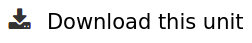# How the rover moves

This unit was carried out jointly by the Physics / Maths teacher and the Mechanical construction teacher.
This will allow students to learn and understand how does the rover moves, through experimentation and analysis.
To do this, the students will therefore need to mobilize and combine knowledge and skills from different subjects such as Maths, Physics and mechanics rules.

The first step will be to show to the students the differents motions used with the remote control.
Then, they will learn how many motors is used, the revolution direction of each motors for the differents motions (forward, turn left…).
They sum up the result on a slide which could be used as a rover used notice.

The second part of the unit will be the understanding of gear principle, transmission ratio, output speed in function of input speed. The student must find the speed wheel tracks. By experimentation, students will verify or compare speed result.
At least, students will be able to calculate the rover speed, to learn which parameters can change this speed. They can compare this result to real rover speed easily.